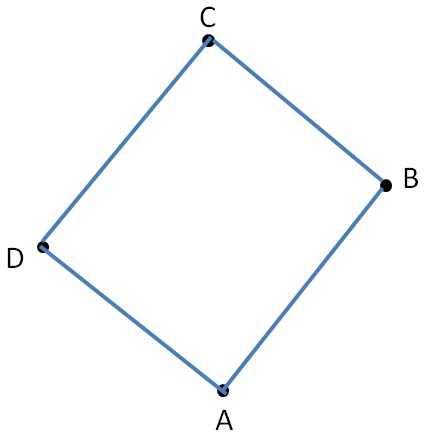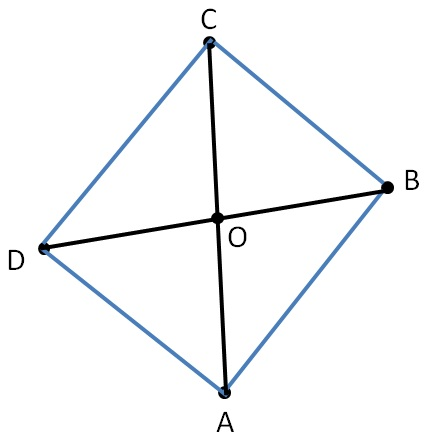A 4-sided polygon is a quadrilateral

Polygon

Polygon is a simple closed figure made of line segments• 4 vertices − A, B, C, D
• 4 sides − AB, BC, CD, DA
• 4 angles − ∠A, ∠B, ∠C, ∠D

Opposite sides

• AB & CD are opposite sides
• AD & BC are opposite sides

• AB, BC are adjacent sides
• BC, CD are adjacent sides
• CD, DA are adjacent sides"Opposite angles

• ∠A, ∠C are opposite angles
• ∠B, ∠D are opposite angles• ∠A, ∠B are adjacent angles.
• ∠A, ∠D are adjacent angles.
• ∠B, ∠C are adjacent angles.
• ∠C, ∠D are adjacent angles.Line joining two opposite vertices of a quadrilateral is a diagonal.

Here, AC, BD are diagonals of the quadrilateral.

And they meet at point O.

Subscribe to our Youtube Channel - https://you.tube/teachoo

1. Chapter 4 Class 6 Basic Geometrical Ideas
2. Concept wise